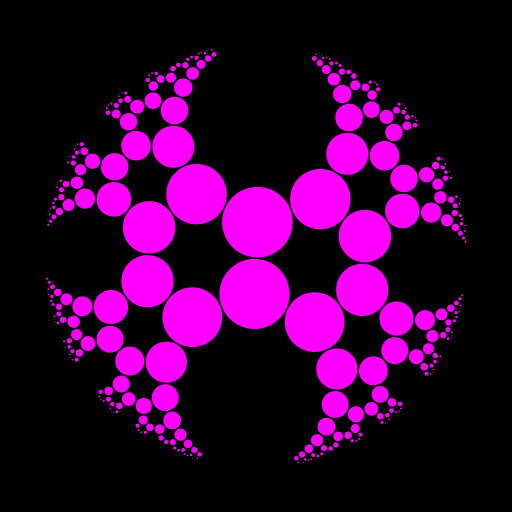Want to share your content on R-bloggers? click here if you have a blog, or here if you don't.

I came across this interesting paper entitled Complex Variables Visualized and written by Thomas Ponweiser.

In particular, I was intrigued by the generalized powers of a Möbius transformation (of a matrix, actually), and their actions on the modular tessellation.

So I firstly implemented the generalized powers in my package PlaneGeometry. Then I wrote the script below to visualize the orbit of the modular tessellation under the action of $$R^t$$, $$0 \leqslant t < 3$$, with the notations of the paper. The command fplot(u) generates the modular tessellation under the action of $$R^t$$ when u is the value of $$t$$. Then I use the gifski package to create the animation.

To get the modular transformations $$z \mapsto \frac{az+b}{cz+d}$$, I use the unimodular function of the elliptic package. It generates the quadruples $$(a,b,c,d)$$ of positive integers such that $$ad-bc=1$$. Then we can get all such quadruples $$(a,b,c,d) \in \mathbb{Z}^4$$ by inverting these modular transformations, swapping $$a$$ and $$d$$ and changing their signs.

library(PlaneGeometry)
library(elliptic) # for the 'unimodular' function

# Möbius transformations
T <- Mobius$new(rbind(c(0,-1), c(1,0))) U <- Mobius$new(rbind(c(1,1), c(0,1)))
R <- U$compose(T) # R^t, generalized power Rt <- function(t) R$gpower(t)

# starting circles
I <- Circle$new(c(0,1.5), 0.5) TI <- T$transformCircle(I)

# modified Cayley transformation
Phi <- Mobius$new(rbind(c(1i,1), c(1,1i))) # plotting function #### n <- 8L transfos <- unimodular(n) fplot <- function(u){ opar <- par(mar = c(0,0,0,0), bg = "black") plot(NULL, asp = 1, xlim = c(-1.1,1.1), ylim = c(-1.1,1.1), xlab = NA, ylab = NA, axes = FALSE) draw(unitCircle, col = "black") for(i in 1L:dim(transfos)[3L]){ transfo <- transfos[,,i] # M <- Mobius$new(transfo)
draw(M$compose(Rt(u))$compose(Phi)$transformCircle(I), border = "black", col = "magenta") draw(M$compose(Rt(u))$compose(Phi)$transformCircle(TI),
border = "black", col = "magenta")
M <- M$inverse() draw(M$compose(Rt(u))$compose(Phi)$transformCircle(I),
border = "black", col = "magenta")
draw(M$compose(Rt(u))$compose(Phi)$transformCircle(TI), border = "black", col = "magenta") # diag(transfo) <- -diag(transfo) M <- Mobius$new(transfo)
draw(M$compose(Rt(u))$compose(Phi)$transformCircle(I), border = "black", col = "magenta") draw(M$compose(Rt(u))$compose(Phi)$transformCircle(TI),
border = "black", col = "magenta")
M <- M$inverse() draw(M$compose(Rt(u))$compose(Phi)$transformCircle(I),
border = "black", col = "magenta")
draw(M$compose(Rt(u))$compose(Phi)$transformCircle(TI), border = "black", col = "magenta") # d <- diag(transfo) if(d[1L] != d[2L]){ diag(transfo) <- rev(d) M <- Mobius$new(transfo)
draw(M$compose(Rt(u))$compose(Phi)$transformCircle(I), border = "black", col = "magenta") draw(M$compose(Rt(u))$compose(Phi)$transformCircle(TI),
border = "black", col = "magenta")
M <- M$inverse() draw(M$compose(Rt(u))$compose(Phi)$transformCircle(I),
border = "black", col = "magenta")
draw(M$compose(Rt(u))$compose(Phi)$transformCircle(TI), border = "black", col = "magenta") } } for(i in 1L:dim(transfos)[3L]){ transfo <- transfos[,,i] # M <- Mobius$new(transfo)$compose(T) draw(M$compose(Rt(u))$compose(Phi)$transformCircle(I),
border = "black", col = "magenta")
draw(M$compose(Rt(u))$compose(Phi)$transformCircle(TI), border = "black", col = "magenta") M <- M$inverse()
draw(M$compose(Rt(u))$compose(Phi)$transformCircle(I), border = "black", col = "magenta") draw(M$compose(Rt(u))$compose(Phi)$transformCircle(TI),
border = "black", col = "magenta")
#
diag(transfo) <- -diag(transfo)
M <- Mobius$new(transfo)$compose(T)
draw(M$compose(Rt(u))$compose(Phi)$transformCircle(I), border = "black", col = "magenta") draw(M$compose(Rt(u))$compose(Phi)$transformCircle(TI),
border = "black", col = "magenta")
M <- M$inverse() draw(M$compose(Rt(u))$compose(Phi)$transformCircle(I),
border = "black", col = "magenta")
draw(M$compose(Rt(u))$compose(Phi)$transformCircle(TI), border = "black", col = "magenta") # d <- diag(transfo) if(d[1L] != d[2L]){ diag(transfo) <- rev(d) M <- Mobius$new(transfo)$compose(T) draw(M$compose(Rt(u))$compose(Phi)$transformCircle(I),
border = "black", col = "magenta")
draw(M$compose(Rt(u))$compose(Phi)$transformCircle(TI), border = "black", col = "magenta") M <- M$inverse()
draw(M$compose(Rt(u))$compose(Phi)$transformCircle(I), border = "black", col = "magenta") draw(M$compose(Rt(u))$compose(Phi)$transformCircle(TI),
border = "black", col = "magenta")
}
}
}

# animation ####
library(gifski)
u_ <- seq(0, 3, length.out = 181L)[-1L]
save_gif({
for(u in u_){
fplot(u)
}
}, "ModularTessellation.gif", 512, 512, delay = 1/12, res = 144)